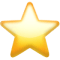Search app# FinCalcPro Financial Solver

(4)
Price
\$2.99
Category
Last Update
Jun 04, 2021
View in store

#### Store Performance Index

64%

Store Performance Index shows overall performance of your app on app stores. It is based on combined ASO & reviews metrics.

See more data

## Ratings & Reviews performance for FinCalcPro Financial Solver

Ratings & Reviews performance provides an overview of what users think of your app. Here are the key metrics to help you identify how your app is rated by users and how successful is your review management strategy.

Number of reviews,
total

4

Avg rating,
total2.0

#### Description

1984 chars

Financial mathematics calculator, very easy to use. FinCalcPro CAN SOLVE EVERY VARIABLE OF THE ABOVE LIST !!! The App can solve equations. In the screenshots the user input is black, the calculated data are red. The following types of calculation are supported: • PERCENTAGES - calculation of the percentage (augmentation / reduction) - calculation of the previous value - calculate of the new value • LINEAR INTEREST - calculation of the initial capital - calculation of the end capital - calculation of the interest - calculation of the period • COMPOUND INTEREST - calculation of the initial capital - calculation of the end capital - calculation of the interest - calculation of the period • INTRA-YEAR COMPOUNDING - calculation of the initial capital - calculation of the end capital - calculation of the interest - calculation of the period - calculation of the number of intra-year periods -conversion nominal interest / effective interest - conversion effective interest / nominal interest • CONTINUOUSLY COMPOUNDED INTEREST - calculation of the initial capital - calculation of the end capital - calculation of the interest - calculation of the period -conversion continuous interest rate / effective interest rate - conversion effective interest rate / continuous interest rate • ORDINARY ANNUITIES AND ANNUITIES DUE - calculation of the annuity - calculation of the interest - calculation of the final value - calculation of the present value • PERPETUITIES - calculation of the annuity - calculation of the interest - calculation of the present value • LINEAR DEPRECIATION - calculation of the depreciation rate - calculation of the new value - calculation of the residual value • DEGRESSIVE DEPRECIATION - calculation of the depreciation rate - calculation of the new value - calculation of the residual value • NPV - IRR - calculation of the Net Present Value (NPV) - calculation of the Internal Rate of Return (IRR) - calculation of the Profitability Index

User reviews affect conversion to installs and app rating. Featured and helpful reviews are the first to be noticed by users and in case of no response can affect download rate. This is why it is highly recommended to reply to them.

Makita_K
Jan 12, 2020

Can’t change language.

Andrey AV
May 09, 2017

Can't change language on EnglishDeveloped by Francesco Grassi.

FinCalcPro Financial Solver is ranking in   Finance & Business

Last update was at Jun 04, 2021and the current version is 2.6.

FinCalcPro Financial Solver have a 4a user reviews.

FinCalcPro Financial Solver was released in the App Store. It is developed by Francesco Grassi, who have also released the following apps.

FinCalcPro Financial Solver has 4 user reviews.​

FinCalcPro Financial Solver has an average rating of 2.0. ​The latest version of FinCalcPro Financial Solver 2.6​ ​​was ​released on Jun 04, 2021​.